CAT  >  Practice Questions Level 2: Matrix

# Practice Questions Level 2: Matrix - Notes | Study Level-wise Practice Questions for CAT Preparation - CAT

 1 Crore+ students have signed up on EduRev. Have you?

This EduRev document offers 10 Multiple Choice Questions (MCQs) from the topic Number System (Level - 2). These questions are of Level - 2 difficulty and will assist you in the preparation of CAT & other MBA exams. You can practice/attempt these CAT Multiple Choice Questions (MCQs) and check the explanations for a better understanding of the topic.

Question for Practice Questions Level 2: Matrix
Try yourself:Directions: In the following question, find out which of the answer figures (a, b, c and d) completes the given figure matrix.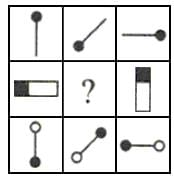Question for Practice Questions Level 2: Matrix
Try yourself:Directions: In the following question, find out which of the answer figures (a, b, c and d) completes the given figure matrix.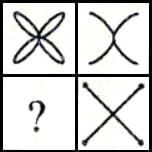Question for Practice Questions Level 2: Matrix
Try yourself:Directions: In the following question, find out which of the answer figures (a, b, c and d) completes the given figure matrix.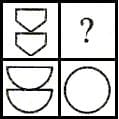Question for Practice Questions Level 2: Matrix
Try yourself:Directions: In the following question, find out which of the answer figures (a, b, c and d) completes the figure matrix.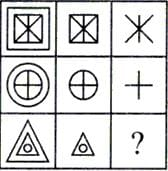Question for Practice Questions Level 2: Matrix
Try yourself:Directions: In the following question, find out which of the answer figures (a, b, c and d) completes the figure matrix.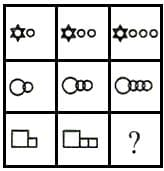Question for Practice Questions Level 2: Matrix
Try yourself:Directions: In the following question, which of the answer figures (a, b, c and d) completes the figure matrix.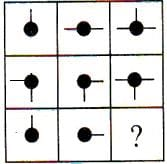Question for Practice Questions Level 2: Matrix
Try yourself:Directions: In the following question, find out which of the answer figures (a), (b), (c) and (d) completes the figure matrix.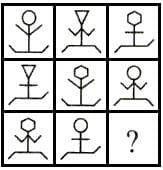Question for Practice Questions Level 2: Matrix
Try yourself:Directions: In the following question, find out which of the answer figures (a), (b), (c) and (d) will complete the figure matrix.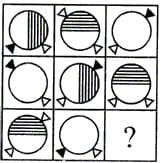Question for Practice Questions Level 2: Matrix
Try yourself:Directions: In the following question, find out which of the answer figures (a), (b), (c) and (d) completes the figure matrix.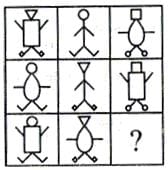Question for Practice Questions Level 2: Matrix
Try yourself:Directions: In the following question, find out which of the answer figures (a, b, c and d) completes the figure matrix.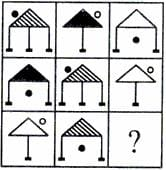The document Practice Questions Level 2: Matrix - Notes | Study Level-wise Practice Questions for CAT Preparation - CAT is a part of the CAT Course Level-wise Practice Questions for CAT Preparation.
All you need of CAT at this link: CAT

## Level-wise Practice Questions for CAT Preparation

277 docs
 Use Code STAYHOME200 and get INR 200 additional OFF

## Level-wise Practice Questions for CAT Preparation

277 docs

### How to Prepare for CAT

Read our guide to prepare for CAT which is created by Toppers & the best Teachers

Track your progress, build streaks, highlight & save important lessons and more!

,

,

,

,

,

,

,

,

,

,

,

,

,

,

,

,

,

,

,

,

,

;